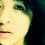## Monday, April 06, 2009

### Those Question Marks Need to be Removed

What number should logically replace the ?
4 5 9 4 2
8 6 2 6 4
2 3 ? 2 7
7 1 2 3 5
3 8 3 6 2

1.2.Counting columns

2+4+7+5+2 = 20
4+6+2+3+6 = 21
9+2+?+2+7 should be 22
5+6+3+1+8 = 23
4+8+2+7+3 = 24

solving 9+2+?+2+7 = 22
? = 6

3.Counting columns

2+4+7+5+2 = 20
4+6+2+3+6 = 21
9+2+?+2+7 should be 22
5+6+3+1+8 = 23
4+8+2+7+3 = 24

solving 9+2+?+2+7 = 22
? = 6

4.Leave your answer or, if you want to post a question of your own, send me an e-mail. Look in the about section to find my e-mail address. If it's new, I'll post it soon.

Please don't leave spam or 'Awesome blog, come visit mine' messages. I'll delete them soon after.

Enter your Email and join hundreds of others who get their Question of the Day sent right to their mailbox

The Lamplight Manor Puzz 3-D